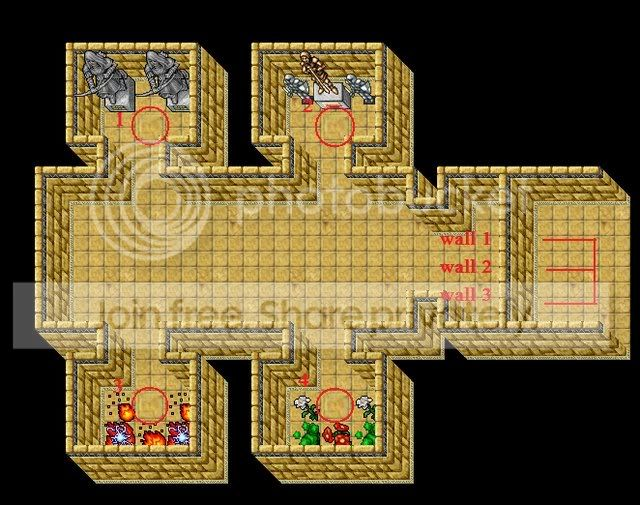# MoveEventMy Special Quest

#### Clary

##### New Member
Hello dear Otland members,
This script was made by a request from Tony
Tony32 said:
This is how it will look like:1. Sorcerer/MS here
2. Druid/ED here
4. Knight/EK here

When all the vocations is on the special square, the three walls, (wall 1,2 and 3) will be removed.
But if someone leaves it's place, the walls will come back.

As you can see, this is a team-work quest.
Credits:
100% by Clary.

Script:
PHP:
``````-- A script made by Clary 100%
function onStepIn(cid, item, pos)
if item.uid == 8001 then

player1pos = {x=1008, y=1038, z=7, stackpos=253}
player1 = getThingfromPos(player1pos)

player2pos = {x=1007, y=1038, z=7, stackpos=253}
player2 = getThingfromPos(player2pos)

player3pos = {x=1006, y=1038, z=7, stackpos=253}
player3 = getThingfromPos(player3pos)

player4pos = {x=1005, y=1038, z=7, stackpos=253}
player4 = getThingfromPos(player4pos)

if player1.itemid > 0 and player2.itemid > 0 and player3.itemid > 0 and player4.itemid > 0 then

player1level = getPlayerLevel(player1.uid)
player2level = getPlayerLevel(player2.uid)
player3level = getPlayerLevel(player3.uid)
player4level = getPlayerLevel(player4.uid)

questlevel = 0

if player1level >= questlevel and player2level >= questlevel and player3level >= questlevel and player4level >= questlevel then

player1voc = getPlayerVocation(player1.uid)
player2voc = getPlayerVocation(player2.uid)
player3voc = getPlayerVocation(player3.uid)
player4voc = getPlayerVocation(player4.uid)

questvoc1 = 1 or 5
questvoc2 = 2 or 6
questvoc3 = 3 or 7
questvoc4 = 4 or 8

if player1voc == questvoc1 and player2voc == questvoc2 and player3voc == questvoc3 and player4voc == questvoc4 then

nwall1pos = {x=1003, y=1038, z=7, stackpos=1}
nwall2pos = {x=1003, y=1037, z=7, stackpos=1}
nwall3pos = {x=1003, y=1036, z=7, stackpos=1}
propos = {x=1008, y=1041, z=7, stackpos=1}
wall1 = getThingfromPos(nwall1pos).uid
wall2 = getThingfromPos(nwall2pos).uid
wall3 = getThingfromPos(nwall3pos).uid

doRemoveItem(wall1)
doRemoveItem(wall2)
doRemoveItem(wall3)
doCreateItem(1112, 1, propos)

doSendMagicEffect(nwall1pos,10)
doSendMagicEffect(nwall2pos,10)
doSendMagicEffect(nwall3pos,10)
end
end
end
return TRUE
end
end

function onStepOut(cid, item, pos)
nwall1pos = {x=1003, y=1038, z=7, stackpos=1}
nwall2pos = {x=1003, y=1037, z=7, stackpos=1}
nwall3pos = {x=1003, y=1036, z=7, stackpos=1}
propos = {x=1008, y=1041, z=7, stackpos=1}
pro1 = getThingfromPos(propos).uid
if getThingfromPos(propos).itemid == 1112 then
doCreateItem(1111, 1, nwall1pos)
doCreateItem(1111, 1, nwall2pos)
doCreateItem(1111, 1, nwall3pos)
doRemoveItem(pro1)
return TRUE
end
end``````
instructions:
Code:
``````~~Start Instructions~~
-put vocquest.lua inside movements/scripts

<movevent event="StepIn" uniqueid="8001" script="vocquest.lua" />
<movevent event="StepOut" uniqueid="8001" script="vocquest.lua" />
~~End Instructions~~
~~Start Changes~~
change those positions to where players should stand

player1pos = {x=1008, y=1038, z=7, stackpos=253}
player2pos = {x=1007, y=1038, z=7, stackpos=253}
player3pos = {x=1006, y=1038, z=7, stackpos=253}
player4pos = {x=1005, y=1038, z=7, stackpos=253}

change this to the level required for this quest
questlevel = 0

change this to the voc required :)
questvoc1 = 1 or 5 -- sorc (pos1)
questvoc2 = 2 or 6 -- druid (pos2)
questvoc3 = 3 or 7 -- paladin (pos3)
questvoc4 = 4 or 8 -- knight (pos4)

position of the walls removed pos (as well created on step out)
nwall1pos = {x=1003, y=1038, z=7, stackpos=1}
nwall2pos = {x=1003, y=1037, z=7, stackpos=1}
nwall3pos = {x=1003, y=1036, z=7, stackpos=1}

position of the wall of check (should be placed somewhere in the map where none see it coz it is just for check)
propos = {x=1008, y=1041, z=7, stackpos=1}
~~End Changes~~``````
Yours,
Clary

Last edited:
•Limos

Sweet script.

#### Amrosh

##### New Member
Clary WOW wonder full idea

#### Clary

##### New Member
Remember Idea credits belong to Tony#### Amrosh

##### New Member
Aha then nice work clary and good idea tony#### Sniperfox

##### New Member
Thanks for releasing it. :thumbup:

#### ivelios

##### New Member
Very interesting quest... Clary you suprised meHmm where is the line with reward? or i must do the chest ;p

#### Clary

##### New Member
Yes u should do the chest well it is easy guysthis is just the hard part of the quest.

#### Kavvson

##### Gdy boli cie glowa wez
Very NiceI use it!

Last edited:

tnnx

#### Inteligentny

##### Im not.
This is what I was looking forthank u
Rep+ mate

#### Inteligentny

##### Im not.
I dont know why but StepOut isn't working :| no errors in console

edit: This one is working:

Code:
``````function onStepOut(cid, item, pos)
nwall1pos = {x=1104, y=1430, z=8, stackpos=1}
nwall2pos = {x=1105, y=1430, z=8, stackpos=1}
nwall3pos = {x=1106, y=1430, z=8, stackpos=1}
propos = {x=1008, y=1041, z=7, stackpos=1}
pro1 = getThingfromPos(propos).uid
doCreateItem(1061, 1, nwall1pos)
doCreateItem(1061, 1, nwall2pos)
doCreateItem(1061, 1, nwall3pos)
return TRUE
end``````
any ideas why the original does not?

#### Grehy

##### Killroy
I dont know why but StepOut isn't working :| no errors in console

edit: This one is working:

Code:
``````function onStepOut(cid, item, pos)
nwall1pos = {x=1104, y=1430, z=8, stackpos=1}
nwall2pos = {x=1105, y=1430, z=8, stackpos=1}
nwall3pos = {x=1106, y=1430, z=8, stackpos=1}
propos = {x=1008, y=1041, z=7, stackpos=1}
pro1 = getThingfromPos(propos).uid
doCreateItem(1061, 1, nwall1pos)
doCreateItem(1061, 1, nwall2pos)
doCreateItem(1061, 1, nwall3pos)
return TRUE
end``````
any ideas why the original does not?
Mine didn't work either, that fixed it right up though, thanks!

very nice quest

#### Elvang

##### Member
That's pretty sweet!

wow awesome ;]

#### KnightmareZ

##### New Member
poor guys who cant do the quest but have to help the other ppl : P

#### taha94

##### New Member
What do i change here?? the first nwall is the position of my walls and what bout the second nwall
Code:
`````` [I]nwall1pos = {x=1026, y=1025, z=8, stackpos=1}
nwall2pos = {x=1026, y=1026, z=8, stackpos=1} <------ position of my walls
nwall3pos = {x=1026, y=1027, z=8, stackpos=1}
propos = {x=1008, y=1041, z=7, stackpos=1}
wall1 = getThingfromPos(nwall1pos).uid
wall2 = getThingfromPos(nwall2pos).uid
wall3 = getThingfromPos(nwall3pos).uid
[/I]
doRemoveItem(wall1)
doRemoveItem(wall2)
doRemoveItem(wall3)
doCreateItem(1112, 1, propos)

doSendMagicEffect(nwall1pos,10)
doSendMagicEffect(nwall2pos,10)
doSendMagicEffect(nwall3pos,10)
end
end
end
return TRUE
end
end

function onStepOut(cid, item, pos)
[I]      nwall1pos = {x=1022, y=1038, z=8, stackpos=1}
nwall2pos = {x=1022, y=1039, z=8, stackpos=1}
nwall3pos = {x=1022, y=1040, z=8, stackpos=1}   <---- What the hell is this
propos = {x=1023, y=1041, z=8, stackpos=1}[/I]
pro1 = getThingfromPos(propos).uid
if getThingfromPos(propos).itemid == 1112 then
doCreateItem(1111, 1, nwall1pos)
doCreateItem(1111, 1, nwall2pos)
doCreateItem(1111, 1, nwall3pos)
doRemoveItem(pro1)
return TRUE
end
end``````

#### vakacjus

##### Lua & C++ Study.
But if someone leaves it's place, the walls will come back.
So we need 5 players for this quest ? ;x